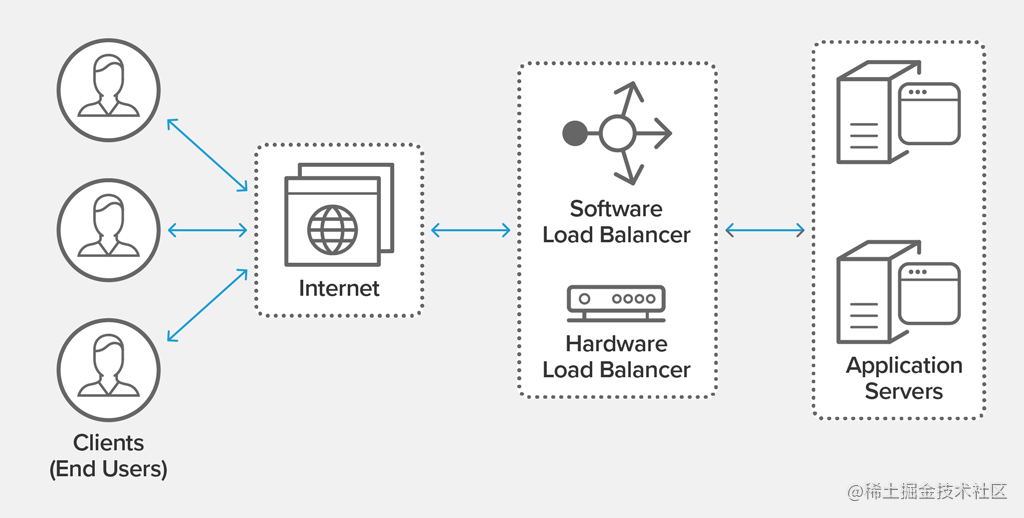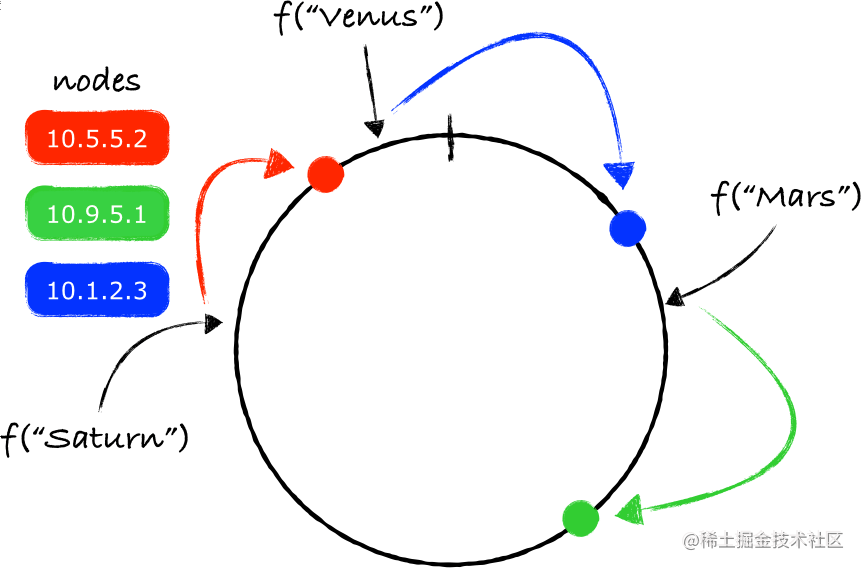# 负载均衡算法之一 - 以 Golang 方式## 基本的负载均衡算法

1. 随机
2. 轮询
3. 最少连接数
4. hash
5. 带权重的轮询

### 前置的介绍

``````type Peer interface {
String() string
}

type Factor interface {
Factor() string
}

type BalancerLite interface {
Next(factor Factor) (next Peer, c Constrainable)
}

type Balancer interface {
BalancerLite
//...more
}

type FactorComparable interface {
Factor
ConstrainedBy(constraints interface{}) (peer Peer, c Constrainable, satisfied bool)
}

type FactorString string

func (s FactorString) Factor() string { return string(s) }

const DummyFactor FactorString = ""

type Constrainable interface {
CanConstrain(o interface{}) (yes bool)
Check(o interface{}) (satisfied bool)
Peer
}

• `Peer` 是一个后端节点。
• 负载均衡器 `Balancer` 持有一组 Peers。
• `Factor``Balancer` 在选取（`Next(factor)`）一个 Peer 时由调度者所提供的参考对象，`Balancer` 可能会将其作为选择算法工作的因素之一。
• 当你身为调度者时，想要调用 Next，却没有什么合适的“因素”提供的话，就提供 `DummyFactor` 好了。
• `Constrainable` 在这组系列文章的最后时分可能会另行介绍，目前将其视而不见就足够了。

### 随机算法 Random

``````package main

import (
"fmt"
"github.com/hedzr/lb/lbapi"
mrand "math/rand"
"sync"
"sync/atomic"
"time"
)

type randomS struct {
peers []lbapi.Peer
count int64
}

func (s *randomS) Next(factor lbapi.Factor) (next lbapi.Peer, c lbapi.Constraintenable) {
l := int64(len(s.peers))
ni := atomic.AddInt64(&s.count, inRange(0, l)) % l
next = s.peers[ni]
return
}

func main() {
lb := &randomS{
peers: []lbapi.Peer{
exP("172.16.0.7:3500"), exP("172.16.0.8:3500"), exP("172.16.0.9:3500"),
},
count: 0,
}

sum := make(map[lbapi.Peer]int)
for i := 0; i < 300; i++ {
p, _ := lb.Next(lbapi.DummyFactor)
sum[p]++
}

for k, v := range sum {
fmt.Printf("%v: %v\n", k, v)
}
}

var seededRand = mrand.New(mrand.NewSource(time.Now().UnixNano()))
var seedmu sync.Mutex

func inRange(min, max int64) int64 {
seedmu.Lock()
defer seedmu.Unlock()
return seededRand.Int63n(max-min) + min
}

type exP string

func (s exP) String() string { return string(s) }

`randomS` 实现了一个微型的、简单的随机算法的 LB。

``````\$ go run ./_examples/simple/random/
172.16.0.8:3500: 116
172.16.0.7:3500: 94
172.16.0.9:3500: 90

#### 正规化

1. 线程安全
2. 可嵌套

``````	l := int64(len(s.peers))
ni := atomic.AddInt64(&s.count, inRange(0, l)) % l
next = s.peers[ni]

``````package random

import (
"github.com/hedzr/lb/lbapi"
mrand "math/rand"
"sync"
"sync/atomic"
"time"
)

var seededRand = mrand.New(mrand.NewSource(time.Now().UnixNano()))
var seedmu sync.Mutex

func inRange(min, max int64) int64 {
seedmu.Lock()
defer seedmu.Unlock()
return seededRand.Int63n(max-min) + min
}

// New make a new load-balancer instance with Round-Robin
func New(opts ...lbapi.Opt) lbapi.Balancer {
return (&randomS{}).init(opts...)
}

type randomS struct {
peers []lbapi.Peer
count int64
rw    sync.RWMutex
}

func (s *randomS) init(opts ...lbapi.Opt) *randomS {
for _, opt := range opts {
opt(s)
}
return s
}

func (s *randomS) Next(factor lbapi.Factor) (next lbapi.Peer, c lbapi.Constraintenable) {
next = s.miniNext()
if fc, ok := factor.(lbapi.FactorComparable); ok {
next, c, ok = fc.ConstraintBy(next)
} else if nested, ok := next.(lbapi.BalancerLite); ok {
next, c = nested.Next(factor)
}
return
}

func (s *randomS) miniNext() (next lbapi.Peer) {
s.rw.RLock()
defer s.rw.RUnlock()

l := int64(len(s.peers))
ni := atomic.AddInt64(&s.count, inRange(0, l)) % l
next = s.peers[ni]
return
}

func (s *randomS) Count() int {
s.rw.RLock()
defer s.rw.RUnlock()
return len(s.peers)
}

func (s *randomS) Add(peers ...lbapi.Peer) {
for _, p := range peers {
}
}

func (s *randomS) AddOne(peer lbapi.Peer) {
if s.find(peer) {
return
}

s.rw.Lock()
defer s.rw.Unlock()
s.peers = append(s.peers, peer)
}

func (s *randomS) find(peer lbapi.Peer) (found bool) {
s.rw.RLock()
defer s.rw.RUnlock()
for _, p := range s.peers {
if lbapi.DeepEqual(p, peer) {
return true
}
}
return
}

func (s *randomS) Remove(peer lbapi.Peer) {
s.rw.Lock()
defer s.rw.Unlock()
for i, p := range s.peers {
if lbapi.DeepEqual(p, peer) {
s.peers = append(s.peers[0:i], s.peers[i+1:]...)
return
}
}
}

func (s *randomS) Clear() {
s.rw.Lock()
defer s.rw.Unlock()
s.peers = nil
}

### 轮询算法 Round-Robin

The term round-robin is derived from the French term ruban, meaning "ribbon". Over a long period of time, the term was corrupted and idiomized to robin.

``````func (s *rrS) miniNext() (next lbapi.Peer) {
ni %= int64(len(s.peers))
next = s.peers[ni]
return
}

s.count 会一直增量上去，并不会取模，这样做的用意在于如果 peers 数组发生了少量的增减变化时，最终发生选择时可能会更模棱两可。

### Hashing 以及 Consistent Hashing

#### 代码实现

``````type Hasher func(data []byte) uint32

// hashS is a impl with ketama consist hash algor
type hashS struct {
hasher   Hasher // default is crc32.ChecksumIEEE
replica  int    // default is 32
hashRing []uint32
keys     map[uint32]lbapi.Peer
peers    map[lbapi.Peer]bool
rw       sync.RWMutex
}

func (s *hashS) Next(factor lbapi.Factor) (next lbapi.Peer, c lbapi.Constraintenable) {
var hash uint32
hash = s.hasher([]byte(factor.Factor()))

ix := sort.Search(len(s.hashRing), func(i int) bool {
return s.hashRing[i] >= hash
})

if ix == len(s.hashRing) {
ix = 0
}

hashValue := s.hashRing[ix]
if p, ok := s.keys[hashValue]; ok {
if _, ok = s.peers[p]; ok {
next = p
}
}

return
}

func (s *hashS) Add(peers ...lbapi.Peer) {
for _, p := range peers {
s.peers[p] = true
for i := 0; i < s.replica; i++ {
hash := s.hasher(s.peerToBinaryID(p, i))
s.hashRing = append(s.hashRing, hash)
s.keys[hash] = p
}
}

sort.Slice(s.hashRing, func(i, j int) bool {
return s.hashRing[i] < s.hashRing[j]
})
}

func (s *hashS) peerToBinaryID(p lbapi.Peer, replica int) []byte {
str := fmt.Sprintf("%v-%05d", p, replica)
return []byte(str)
}

#### 算法思想扼要扫尾keynote，流口水啊，我真的打算去学学了。

### 带权重的轮询算法 Weighted Round-robin

#### Nginx 平滑加权轮询

Nginx 采用一种依据 total 和各个权重之间的差值来平衡选择的方法：每次选择时，都对每个节点的 currentWeight 加上其权重值，然后选择 currentWeight 最大的那个节点，同时该节点的 currentWeight 在减去其权重值复原到增量前。如此反复选择之下，权重大的节点由于增量速度更快而被选中的更多。

``````func (s *wrrS) Next() (best lbapi.Peer) {
total := 0
for _, node := range s.peers {
if node == nil {
continue
}

total += s.mUpdate(node, 0, false)
if s.mTest(best, node) {
best = node
}
}

if best != nil {
s.mUpdate(best, -total, true)
}
return
}

func (s *wrrS) mUpdate(node lbapi.Peer, delta int, success bool) (total int) {
if delta == 0 {
delta = s.m[node].weight
}
s.m[node].current += delta
return s.m[node].weight
}

func (s *wrrS) mTest(best, node lbapi.Peer) bool {
return best == nil || s.m[node].current > s.m[best].current
}

#### LVS 平滑加权轮询

``````/*
Supposing that there is a server set S = {S0, S1, …, Sn-1};
W(Si) indicates the weight of Si;
i indicates the server selected last time, and i is initialized with -1;
cw is the current weight in scheduling, and cw is initialized with zero;
max(S) is the maximum weight of all the servers in S;
gcd(S) is the greatest common divisor of all server weights in S;
*/
while (true) {
i = (i + 1) mod n;
if (i == 0) {
cw = cw - gcd(S);
if (cw <= 0) {
cw = max(S);
if (cw == 0)
return NULL;
}
}
if (W(Si) >= cw)
return Si;
}

• 加权随机算法
• （请求）来源 Hash
• 目的地 Hash
• 加权最少连接数算法
• 等等

:end: